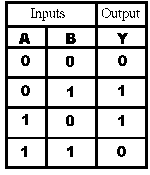# New to QlikView

Discussion board where members can get started with QlikView.

Highlighted
Honored Contributor II

## Xor operator

Hi All,

Please help to provide an example for Xor operator in QV.

Thanks in advance.

1 Solution

Accepted SolutionsMVP & Luminary

## Re: Xor operator

Hi,

Please check the truth table of XOR, if any one value is true (1) then the result is true otherwise false.For example, if I have date like below

Country:

LOAD * INLINE [

Country, Region, Sales

India, APAC, 1000

Singapore , APAC, 500

USA, USA, 600

UK, UK, 300

];

and for the below set analysis expression

=Sum({<Country /= {'India'}>} Sales)

It returns 1400, because for the record with country India /= returns false, because in possible values we have India (Since I do not select any value) and in given set I have India, so both are true then it won't considers this record for calculation.

Suppose if you select India then this expression returns 0.

If I select Singapore then this returns 1500 (500 (Singapore) + 1000 (India)) for

Hope this helps you.

Regards,

Jagan.

4 Replies
Honored Contributor II

## Re: Xor operator

### See this:

What is logic gate (AND, OR, XOR, NOT, NAND, NOR and XNOR)? - Definition from WhatIs.com

### Boolean Operations - C++ Tutorials

Comparison with OR would you clear your concept.

(true OR true) -> true

(true OR false) -> true

(false OR false) -> false

whereas, for XOR

(true XOR true) -> false

(true XOR false) -> true

(false XOR false) -> false

i.e. - ONLY one true is true. (Note: order of true/false would not change the result)

Hope this helps you understand.

Valued Contributor II

## Re: Xor operator

Hi,

A bitwise XOR takes two bit patterns of equal length and performs the logical exclusive OR operation on each pair of corresponding bits. The result in each position is 1 if only the first bit is 1 or only the second bit is 1, but will be 0 if both are 0 or both are 1. In this we perform the comparison of two bits, being 1 if the two bits are different, and 0 if they are the same.

In simple terms, if both conditions are true or false it will give result as " False " . If any one is true you will get result as " True "

Re: Xor Logical Operators

Hope it helps!!

Honored Contributor II

## Re: Xor operator

Thank you for the information.MVP & Luminary

## Re: Xor operator

Hi,

Please check the truth table of XOR, if any one value is true (1) then the result is true otherwise false.For example, if I have date like below

Country:

LOAD * INLINE [

Country, Region, Sales

India, APAC, 1000

Singapore , APAC, 500

USA, USA, 600

UK, UK, 300

];

and for the below set analysis expression

=Sum({<Country /= {'India'}>} Sales)

It returns 1400, because for the record with country India /= returns false, because in possible values we have India (Since I do not select any value) and in given set I have India, so both are true then it won't considers this record for calculation.

Suppose if you select India then this expression returns 0.

If I select Singapore then this returns 1500 (500 (Singapore) + 1000 (India)) for

Hope this helps you.

Regards,

Jagan.